# Velocity - 6th grade (11y) - math problems

#### Number of problems found: 78

• The carThe car has a consumption of 5 liters per 100 km. It has 24 liters of gasoline in the tank. How much petrol remained in the tank when it rides for two and a half hours at a speed of 80 km/h?
• A giraffe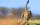A giraffe can run up to 46.93 feet per second. How far could a giraffe run in 1.8 seconds?
• A plane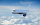A plane is flying at the rate of 350 mph after an eight hour flight is at its destination how far did the plane fly?
• A raindrop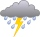A raindrop falls at a rate of 9.3 meters per second (m/s). Use the following facts to convert this speed to kilometers per hour (km/h). 1 km = 1000 m 1 min = 60 sec 1 hour = 60 min
• Water tank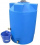The block-shaped tank is 2.5 m long, 100 cm wide and 12 dm high. In how many minutes will it be filled with water to two thirds if 40 liters of water flow into the tank per minute?
• ShortcutThe road from the cottage to the shop 6 km away leads either along a straight road that the bicycle can drive at a speed of 18 km/h or by "shortcut". It measures only 3.6 km. But the road from the cottage is all uphill - at a speed of 8 km/h, you can go h
• Earth rotation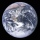How fast is the place on the Earth's equator moving if the Earth's radius is 6378 km?
• The car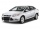The car travels at a speed of 70 km/h, what distance will it travel in 2 hours?
• On a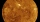On a some day, the Sun, Venus and the Earth are in eclipse, i. E. Venus is between the Sun and the Earth. Venus orbits the Sun in 225 days. In how many years will all three bodies be in alignment again?
• Cyclist 12What is the average speed of a cycle traveling at 20 km in 60 minutes in km/h?
• The swallow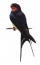The swallow will fly 2.8 km per minute. How many km will the swallow fly in one hour?
• The run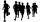On the school playground, the student ran a 60 m track in 9.5 s. What is the average speed of his run?
• I drive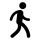I drive 9 km in 30 minutes, what is the average speed of the motion?
• Equal temperature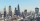The temperatures of the two cities were measured at the same time. The temperature in city A was 60 degrees And rose at a constant rate of 2 degrees per hour. The temperature in city B was 40° and rose at a constant rate of 10° per hour Enter the time in
• A cold front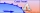At 1:00 PM, the temperature was at 46 degrees. Then, a cold front moved in and decreased the temperature 12 degrees per hour. The temperature at 6:00 PM = _____ degrees.
• Doug bikedDoug biked 5 1/4 miles in 3/4 of an hour. What is his average speed?
• A filterIt is a pool with a volume of 3500 liters. The filter filters at 4m cubic per hour. How many minutes would it filter the entire pool?
• Car loop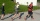The toy car runs at an average speed of 2 m/s. In a minute, it will pass the entire circuit five times. How long is the loop?
• Play tennis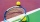Peter and Jirka agreed to go play tennis. Peter's path to the tennis court is 800 meters longer and leads around Jirka's house. Peter comes out first and then picks up Jirka. Together they go at the same average speed as Peter went, so they reach the plac
• Avg speed of flight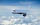The students vice adventure had a 2,367 km flight. If they travel time was 2 hours and 56 minutes, what was their average speed in kilometres per hour?

Do you have an exciting math question or word problem that you can't solve? Ask a question or post a math problem, and we can try to solve it.

We will send a solution to your e-mail address. Solved examples are also published here. Please enter the e-mail correctly and check whether you don't have a full mailbox.

Do you want to convert velocity (speed) units? Velocity - math problems. Examples for 6th grade (the sixth graders).